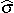## 2. Calculate the estimated standard deviation

The next stage is to calculate the position of the tails of the distribution that has just been drawn. However, in order to calculate the position of the tails, the standard deviation is required. In this version of capability analysis where data has been collected over a period of time, an estimated standard deviation is used. The symbol for the estimated standard deviation is(read “sigma hat”). The formula for the estimated standard deviation is:is calculated when constructing a control chart. Substitute Mforif an X-MR chart has been completed. In the example, the value is 4.653. The denominator (d2) is a weighting factor whose value is based on the subgroup size, n, from the control chart. The value for d2 in the example, based on a subgroup size of 5, is 2.326. A short listing of the d2 values for other subgroup sizes follows. The full table of values is given in the appendix.

 n 2 3 4 5 6 7 8 9 10 d2 1.128 1.693 2.059 2.326 2.534 2.704 2.847 2.97 3.078

The estimated standard deviation for the example is:The estimated standard deviation is calculated to one more decimal place than the original data.

The above article is an excerpt from the “Operational definition” chapter of Practical Tools for Continuous Improvement Volume 2 Statistical Tools.